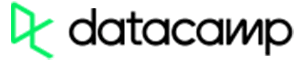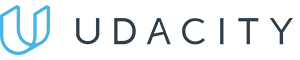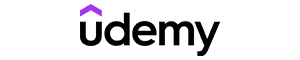Code has been added to clipboard!

# Learn About JavaScript Random Method in a Nutshell

Published Sep 8, 2017
Updated Oct 2, 2019

One of the JavaScript data types is a number. In our previous tutorials, we covered methods used for handling them.

In this tutorial, you will learn all about the JavaScript random method in more detail. We will explain how you can generate random numbers in JavaScript, providing some simple code snippets you can use yourself.

## JavaScript Random: Main Tips

• When returning a random number from 0 (inclusive) to 1 (exclusive), we use Math.random JavaScript method.
• It is a very good idea to create a proper function for Math.random JavaScript method to be used for all random integer purposes.
• Ability to generate random numbers is especially handy when creating games.

## What is JS random?

By default, JavaScript `Math.random()` method will be returning a number higher than 0 (inclusive) and lower than 1 (exclusive):

Example
``Math.random();``

To bring back random integers, we can use `Math.random()` with `Math.floor()`:

Example
``Math.floor(Math.random() * 10);``

Example
``Math.floor(Math.random() * 11);``

Example
``Math.floor(Math.random() * 100);``

Example
``Math.floor(Math.random() * 101);``

Example
``Math.floor(Math.random() * 10) + 1;``

Example
``Math.floor(Math.random() * 100) + 1;``Pros
• Easy to use with a learn-by-doing approach
• Offers quality content
• Gamified in-browser coding experience
• The price matches the quality
• Suitable for learners ranging from beginner to advanced
Main Features
• Free certificates of completion
• Focused on data science skills
• Flexible learning timetablePros
• Simplistic design (no unnecessary information)
• High-quality courses (even the free ones)
• Variety of features
Main Features
• Nanodegree programs
• Suitable for enterprises
• Paid Certificates of completionPros
• Easy to navigate
• No technical issues
• Seems to care about its users
Main Features
• Huge variety of courses
• 30-day refund policy
• Free certificates of completion

## Examples

As we have shown in the examples above, creating a proper function for `random` to be used for all JavaScript random integer purposes is rather useful. Let's see a few more random function JavaScript examples to make sure we covered everything.

The random function JavaScript example below shows a function which always brings back a random number from `min` (included) to `max` (excluded):

Example
``````function getRndInteger(min, max) {
return Math.floor(Math.random() * (max - min) ) + min;
}``````

Now, the next code example shows a function which always brings back a random number from `min` to `max` (both included this time):

Example
``````function getRndInteger(min, max) {
return Math.floor(Math.random() * (max - min + 1) ) + min;
}``````

## JavaScript Random: Summary

• You can use Math.random method to return a random number from 0 (inclusive) to 1 (exclusive).
• Using this method, you can create a proper function using variables to return all JavaScript random integers.
• If you are into creating games, the ability to get random numbers automatically might prove to be extremely useful for you.

Days
Hours
Minutes
Seconds